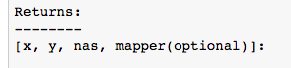# Pandas Categories vs dummies

Jeremy mentioned in class that using pandas categories in Random Forest but using dummy variables in linear regression.

1. Can some explain in more details about the reason again?
2. In R, using factor(categorical variable) has the same result as using dummy variables. What about it in python scikit learn? Do they have same results?
1 Like

A random forest implementation is free to treat categorical and numerical variables differently. I believe Jeremy will suggest using categorical splits for variables with up to five levels and then just using numerical category values beyond that. R does in fact use splitting on categorical values ( equal and not equal versus less than or greater than) but only with cardinality up to 32. Scikit’s random forest implementation simply cannot handle categorical variables. For low cardinality categorical variables, we just convert them to numbers and it works well enough as you can tell from dump truck data.

Linear regression models definitely cannot handle categories and you have to convert them to dummy variables using “one hot encoding”. The difference is that random forests are using less than or equal to comparisons to partition a space whereas linear regression is using the variables as actual values to compute parameters of a known model (a line).

2 Likes

Thank you Terence! Could you please clarify “using categorical splits for variables with up to five levels and then just using numerical category values beyond that.” Do you mean if the character variable has up to 5 levels, we just convert it to pandas categorical variables, then replace them using .cat.codes before running Random Forest Model; and if it has more than 5 levels, we use pd.get_dummies to create dummy columns for each level, and then run Random Forest?

We’ll discuss this in class today.Have all of the values coming into a RF our numbers so there’s no need to convert it to a categorical variable as you ask. The difference between a categorical and numeric value in a RF involves how we split at each node. We exhaustively try all combinations of variables and values within that variable to find a split point four numerical values. Everything less than the value goes one direction everything greater than or equal to goes the other direction down the tree. For categorical variables, if I can remember, we test whether a record has the same value or not the same value. That determines what records we send down the left tree and right tree.

That’s only true if we used dummy values, which we haven’t done so far.

really? Could swear that’s how you told me to handle low cardinality categorical splitting. I’ll have to look back at my Java code.

Yes that’s often a good approach, but it’s not what we’re doing at the moment - we’re simply using the integer codes directly as if they were continuous variables, for all columns.

Hi Everyone
I have a very basic doubt in the same topic. I have observed in the class that jeremy always preprocess the data using proc_df and train_cat functions before splitting it into train and valid. My understanding is that it is required to keep the codes consistent in both the datasets.
However, I am confused about how to handle the test data. Should we combine it with the training for preprocessing (by preprocessing I mean converting categorical to numerical codes using .cat.codes +1) and then split it back into train and test or do we process them separately. My only concern is that if we process the training and test files separately, will that lead to different numerical codes for the same categories within a column?
Correct me if my understanding is wrong @jeremy . Thanks!

I’m not sure but when you apply proc_df on the train data, it output something as mapper which you can store and when you want to apply the same transformation on test you just pass the same mapper to proc_df. This is from the description of proc_df. @jeremy correct me if I’m wrongGreat question! We use `apply_cats` for the test set, to handle this problem. That way, the train and test sets have the same categorical mappings.

That’s also a good point - but note that this is for normalizing continuous vars, not for categorical vars.

1 Like

Thanks!